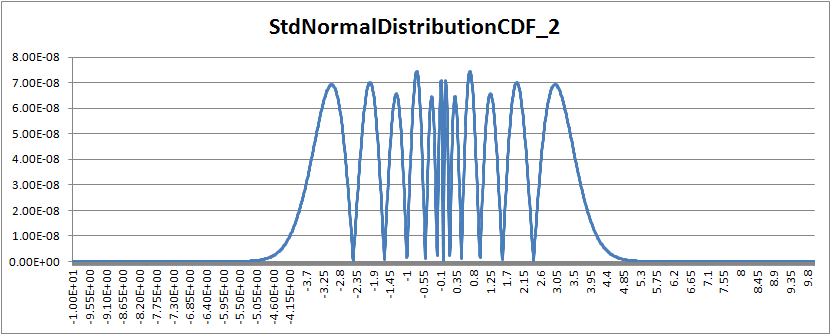## 12 September 2012

### Part 4: T-SQL Implementation of NORMDIST / NORM.S.DIST

This is Part 4 of a set of posts. The other posts are:
Part 1: Introduction
Part 2: Probability Density Function Implementation
Part 3: Cumulative Distribution Function Implementation 1
Part 4: Cumulative Distribution Function Implementation 2
Part 5: Cumulative Distribution Function Implementation 3
Part 6: References and Notes

# Cumulative Distribution Function Implementation 2

The cumulative distribution function has no closed form expression and can only be approximated (see Part 1.)
The following implementation uses approximation 26.2.17 from Abramowitz & Stegun (1964) (see Part 6) for full references.

-- =============================================
-- Author:    Eli Algranti
-- Description:  Standard Normal Distribution
--        Cummulative Distribution Function
--        using polinomial approximation 26.2.17
--        from Abramowitz & Stegun (1964)
-- Remarks:    This function depends on
--        [dbo].[StdNormalDistributionPDF]
--        to calculate the Standard Normal
--        Distribution PDF for the value
-- Copyright:  Eli Algranti (c) 2012
--
-- This code is licensed under the Microsoft Public
-- =============================================
CREATE FUNCTION [dbo].[Stdnormaldistributioncdf_2] (@x FLOAT)
returns FLOAT
AS
BEGIN
DECLARE @Z FLOAT = Abs(@x)

IF ( @Z >= 15 ) -- value is too large no need to compute
BEGIN
IF @x > 0
RETURN 1;

RETURN 0;
END

-- Compute the Standard Normal Cummulative Distribution using
-- polinomial approximation 26.2.17 from Abramowitz & Stegun (1964)
DECLARE @p FLOAT = 0.2316419;
DECLARE @b1 FLOAT = 0.319381530;
DECLARE @b2 FLOAT = -0.356563782;
DECLARE @b3 FLOAT = 1.781477937;
DECLARE @b4 FLOAT = -1.821255978;
DECLARE @b5 FLOAT = 1.330274429;
DECLARE @t FLOAT = 1.0 / ( 1.0 + @p * @Z );
-- For efficiency compute sequence of powers of @t (instead of calling POWER(@t,2), POWER(@t,3), etc.)
DECLARE @t2 FLOAT = @t * @t;
DECLARE @t3 FLOAT = @t2 * @t;
DECLARE @t4 FLOAT = @t3 * @t;
DECLARE @cd FLOAT = 1.0 - [dbo].[Stdnormaldistributionpdf](@Z) * (
@b1 * @t + @b2 * @t2 + @b3 * @t3 + @b4 * @t4 +
@b5 *
@t4 * @t )

IF @x > 0
RETURN @cd;

RETURN 1.0 - @cd;
END

### Verification Using Excel 2010 as Reference

Maximum difference 7.4506132929919E-08 at value 0.719999999999832### Verification Using Excel 2007 as Reference

Maximum difference 7.4506132929919E-08 at value 0.719999999999832### Discussion

This approximation provides a precision of eight decimal places and surprisingly the same maximum difference for both versions of Excel. I had to check twice.
Unlike the previous implementation this one is not a pure polynomial approximation and requires the PDF to be computed.
The performance of this implementation can be vastly improved if the PDF needs to be computed anyway by passing the pre-computed PDF value as a parameter instead of computing it in the function.